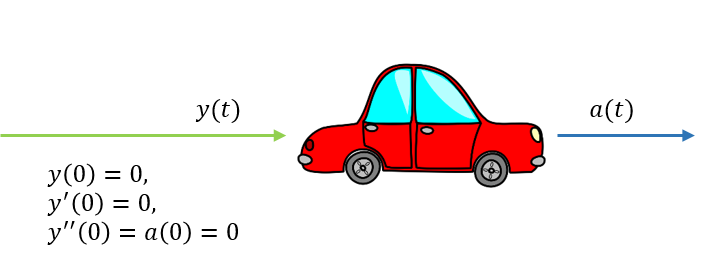# Classical control: Transfer functions

Friday, Sep 21, 2018
Section: Home / Post / Control systems
Categories: Engineering,
Tags: Matlab, Control Systems,

A transfer function relates the output of a system to its input when it is represented in the Laplace domain. An assumption is made that initial steady-state response is 0. If $Y(s)$ is the output of a system, $X(s)$ is the input, then the transfer function is:

$$H(s) = \frac{Y(s)}{X(s)}$$

### Example - A CarA car as a system: The input is the acceleration. The output is the total distance travelled. Initial conditions are zero.

Consider a car as a system. The input $a(t)$ to the car is the acceleration at each time instant. The output $y(t)$ is the total distance it travelled from the start. The system can be described in the time domain as:

Integrating acceleration gives velocity, and integrating velocity gives distance travelled. Taking the Laplace transform using the table of transforms gives:

Thus, the transfer function becomes:

A transfer function can be created in MatLab using:

s = tf('s');    % define the s-domain variable as a symbol
H = 1 / s^2;    % define transfer function in terms of symbol


## Response - Frequency Domain

A system’s response is the output in reaction to input in the time domain. In the frequency domain, the response is a product of the transfer function and the input:

$$Y(s) = H(s) \cdot X(s)$$

There are several standard inputs used to illustrate system responses.

### Impulse Response

An impulse input, $\delta(t)$ is zero everywhere except $t=0$ where $\delta(0)=\infty$. By definition $\int{\delta(t)dt} = 1$ and $\mathcal{L}(\delta(t)) = 1$. The impulse response shows how a system reacts to an instantaneous input.

For the car example, the impulse response i.e. the distance travelled in response to an instantaneous, fleeting infinite acceleration at $t=0$ is:

$$Y(s) = H(s) \cdot \mathcal{L}(\delta(t)) = H(s) \cdot 1 = \frac{1}{s^2}$$

impulseplot(H, 10);


The impulse response for $a(t) = \delta(t)$ can be verified in the time domain as well:

### Step Response

A step input, $u(t)$ is zero for $t < 0$ and one for $t \ge 0$. By definition $\mathcal{L}(u(t)) = s$. The unit response shows how a system reacts to a steady constant input.

For the car example, the step response i.e. the distance travelled in response to a constant acceleration of $1$ starting at $t=0$ is:

$$Y(s) = H(s) \cdot \mathcal{L}(u(t)) = H(s) \cdot s = \frac{1}{s}$$

stepplot(H, 10);


The step response for $a(t) = u(t)$ can be verified in the time domain as well:

## Response - Time Domain

In the time domain, response calculation is more complicated. The response is the convolution between the impulse response $h(t)$ and the input $x(t)$ in time. The impulse response is the transfer function equivalent in the time domain.

## Poles and Zeros

The transfer function scales input to output. It stands to reason that an infinite scaling of a finite input is undesirable and indicates an unstable system. Similarly, a zero scaling indicates a damped/dissipative system which may or may not be desirable. The form of a transfer function is:

$$H(s) = \frac{N(s)}{D(s)}$$

Where $N$ is the numerator and $D$ is the denominator. A system will be unstable when $D(s) = 0$. These points are known as poles. Conversely, a system’s response will tend to $0$ when $N(s) = 0$. These points are known as zeros.

Recall that $s = \sigma + \iota \omega$. And that in the laplace domain, signals are represented as a combination of decaying sinusoids i.e. $e^{-st}$ for different values of $s$. So for $s=0 \rightarrow e^{-st} = 1$ is constant. For $s=1 + \iota \rightarrow e^{-t}e^{-\iota t}$ is a decaying sinusoid.

For the car example, the transfer function is $\frac{1}{s^2}$. Poles occur at $s=0$. There are no zeros. This makes sense. A zero frequency variable means constant input i.e. acceleration. Any constant acceleration will cause the response i.e. distance travelled to go to infinity over time. Any other values of $s = \pm \sigma \pm \iota \omega$ will mean that the acceleration will oscillate between positive and negative values that are continuously growing/decaying. So the distances travelled forwards and backwards will never exactly cancel out to $0$ nor will they reach infinity.

The poles and zeros give valuable information about what frequencies of inputs produce what results. Poles indicate resonant frequencies and must be avoided. Any phisically realizable system must have more zeros than poles. If the system had more poles, it would just magnify responses to all frequencies except for zeros:

Meaning that over time, the system response would go to infinity.